# Gravity Field Stress

The Gravity field stress option is used to define an in-situ stress field which varies with depth. Gravity field stress is typically used for surface or near surface excavations.

The depth can be measured from either:

• A user-specified Ground Surface Elevation (useful if the ground profile is a single horizontal elevation).
• The actual ground surface of your model (useful if the ground profile has a variable elevation).

The depth and the unit weight of overlying material determines the vertical stress distribution throughout the model. The horizontal stresses are calculated by multiplying the vertical stress by the Horizontal/Vertical Stress Ratios.

In the Field Stress dialog, the following parameters are used to define a Gravity stress field.

## Ground Surface Elevation

The Ground Surface Elevation is the vertical (y) coordinate of the ground surface with respect to your coordinate system. It is NOT the depth below ground surface of your excavations!

• A horizontal dotted line marking the Ground Surface Elevation will automatically be displayed on the model. The display of this line can be toggled on or off in the View menu.
• If you have selected the Use Actual Ground Surface check box, the Ground Surface Elevation option is not applicable, and will be disabled. See below for more information.

## Unit Weight of Overburden

The Unit Weight Of Overburden is an AVERAGE value of the unit weight of all materials in your model. It is used in conjunction with the Ground Surface Elevation to calculate the vertical component of the gravitational field stress at any point in the model.

• If you have selected the Use Actual Ground Surface check box, the Unit Weight of Overburden option is not applicable, and will be disabled. See below for more information.

## Use Actual Ground Surface

If the Use Actual Ground Surface check box is selected:

• The initial vertical stress at a given point is calculated using the depth below the actual ground surface of the model, and the actual unit weight of the overlying material(s) as entered in the Define Material Properties dialog.
• The Ground Surface Elevation and Unit Weight of Overburden options will be disabled.

The Use Actual Ground Surface option can be useful for surface excavations such as open pit mines, slopes, rock cuts etc. The advantage of using this option, is that it provides a better initial estimate of the vertical in-situ stress, for surface excavations where the ground surface is irregular, and is not defined by a single horizontal surface.

The Use Actual Ground Surface option is also recommended for use in conjunction with the Shear Strength Reduction stability analysis option. In general, it provides more reliable and accurate results with less computation, due to the more realistic estimate of the initial stress distribution.

## Use Effective Stress Ratio

The Use Effective Stress Ratio check box allows you to specify whether the Horizontal / Vertical stress ratios are applied to the Total vertical stress or the Effective vertical stress, when calculating the horizontal stress.

See below for the equations which are used in both cases.

## Use Variable Stress Ratio

By default the horizontal / vertical stress ratio K is a constant value (i.e. horizontal stress is directly proportional to the vertical stress).

The Use Variable Stress Ratio check box allows you to define a variable stress ratio which is a function of depth z according to the following equation:

K = a + bzc

where:

K = horizontal / vertical stress ratio

z = depth from ground surface

a, b, c = user-defined parameters

If you select this check box you can enter values of a, b and c to define the stress ratio as a function of depth z according to this equation.

## Stress Ratios

The Horizontal / Vertical stress ratios (In-Plane and Out-Of-Plane) are used to calculate the horizontal components of the gravitational field stress, based on the vertical stress at any point in the model.

• The stress ratios which you enter will apply to either the Total stress or Effective stress, according to the selection of the Use Effective Stress Ratio check box (see above).
• If the Use Variable Stress Ratio check box is selected then the stress ratios can be defined as a function of depth (see above).

If the Use Effective Stress Ratio check box is NOT selected, then the horizontal stress is calculated from the total vertical stress, as follows: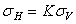where: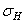= total horizontal stress= total vertical stress= horizontal/vertical stress ratio (total stress)

TIP: the Stress Block indicates the relative magnitude and direction of the In-Plane principal field stresses. For a Gravity field stress, the Stress Block is always oriented with the vertical and horizontal axes. If the Stress Block looks wrong then check the value of the In-Plane Horizontal / Vertical Stress Ratio.

If the Use Effective Stress Ratio check box IS selected, then the horizontal stress is calculated from the effective vertical stress, as follows: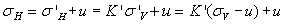where:= total horizontal stress= effective horizontal stress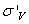= effective vertical stress= total vertical stress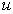= pore pressure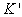= horizontal/vertical stress ratio (effective stress)

Stress Ratio for Soils

See the RS2 Theory section for a document (In-situ Stress States in Soils) which discusses the horizontal/vertical effective stress ratio for soils (the coefficient of earth pressure at rest), and various formulae which can be used to estimate a value of K for normally consolidated or over consolidated soils.

Variable Stress Ratio

If the Use Variable Stress Ratio check box is selected then the stress ratios can be defined as a function of depth, see above for details.

## Locked In Stress

You may also include Locked In components of horizontal stress in the In Plane and / or Out-Of-Plane directions. The Locked In stress is simply the horizontal stress at the ground surface. (In most cases we assume this is zero).

This fixed component of horizontal stress is added to the horizontal stress calculated from the vertical stress and the Horizontal/Vertical Stress Ratio, to determine the total horizontal stress at any point.

## Custom Field Stress

Customized field stress may be defined for individual materials in a model, by selecting the Advanced button, and defining custom field stress as required. See the Custom Field Stress topic for details.